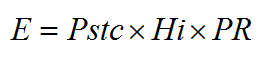Principle Formula Calculator

# Calculation of solar photovoltaic power and energy.

## Principle

The principle of solar photovoltaic is to convert solar energy of light (photons) into electricity. When photons heat special materials they create a displacement of electrons that generate a continuous current. Solar cells are connected in series to form photovoltaic panels that are connected together to crate a PV generator. This generator can be connected to an inverter to transform continuous current in alternative current 3-phase or single phase and connected to the grid or to a storage system.

## Formula to calculate PV energy

How to calculate annual output energy of a solar photovoltaic (PV) system? The simplest formula is :Where :
E = electric energy PV production (kWh/year)
Hi = global incident radiation (kWh/m²/year)
Pstc = sum of peak power at STC conditions of photovoltaic solar panels (kWp)
PR = Performance ratio of the solar PV system (without unit)

## Calculator : solar PV energy and financial gain

Enter your own values in the white boxes, results are displayed in the green boxes.

Power of solar panels, Pstc : kWp
Global incident radiation, Hi :   kWh/m²/year
Performance ratio, PR :   without unit
The performance ratio include all losses of the photovoltaic solar system : temperature derating, inverter yield, losses in cables, losses due to snow and smear and dust... A typical value of PR is between 0.7 and 0.8.
Average annual energy in output of solar PV generator :     kWh/year
MWh/year
Currency
Cost of energy :  /kWh
Total annual amount of electricity bill : /year